How Pooling layer helps in reducing dimension in convolutional neural networks?

One of the most important layer in a CNN architecture is Pooling layer. In this article we will understand what is pooling layer? what does pooling layer do? and how pooling layer works? we will also look at different types of pooling such as max pooling, average pooling and global average pooling.Photo by Andras Kerekes

What is Pooling layer and what does pooling layer do?

In simple words Pooling is used for dimensionality reduction in CNN. Why dimensionality reduction? For decreasing the computational power required to process the data. But pooling is not just for reducing the dimension only, it also helps in extracting the dominant features like edges in the image.

How pooling layer works?

So, now we know that pooling is used for dimensionality reduction but how pooling reduces dimension? Pooling works similar to filters. Consider the below image where we are using a filter of size 2 X 2. In case of filters, we used to multiply filter values to the input element wise and calculate the sum. In case of pooling, we still have the filter window but instead of multiplying we do some operation like taking max or average within the window. For example, in the below image having a 2 X 2 filter with stride 2, we have taken the max of the filter window and taken it as the activation function. Consider the green area where we have taken the max of the 4 values that is 7 and took it as the activation function then filter moves to the next position that is the red area and again it took the maximum value that is 10. Similarly, the filter moves to the orange and blue area and takes the max value from those areas.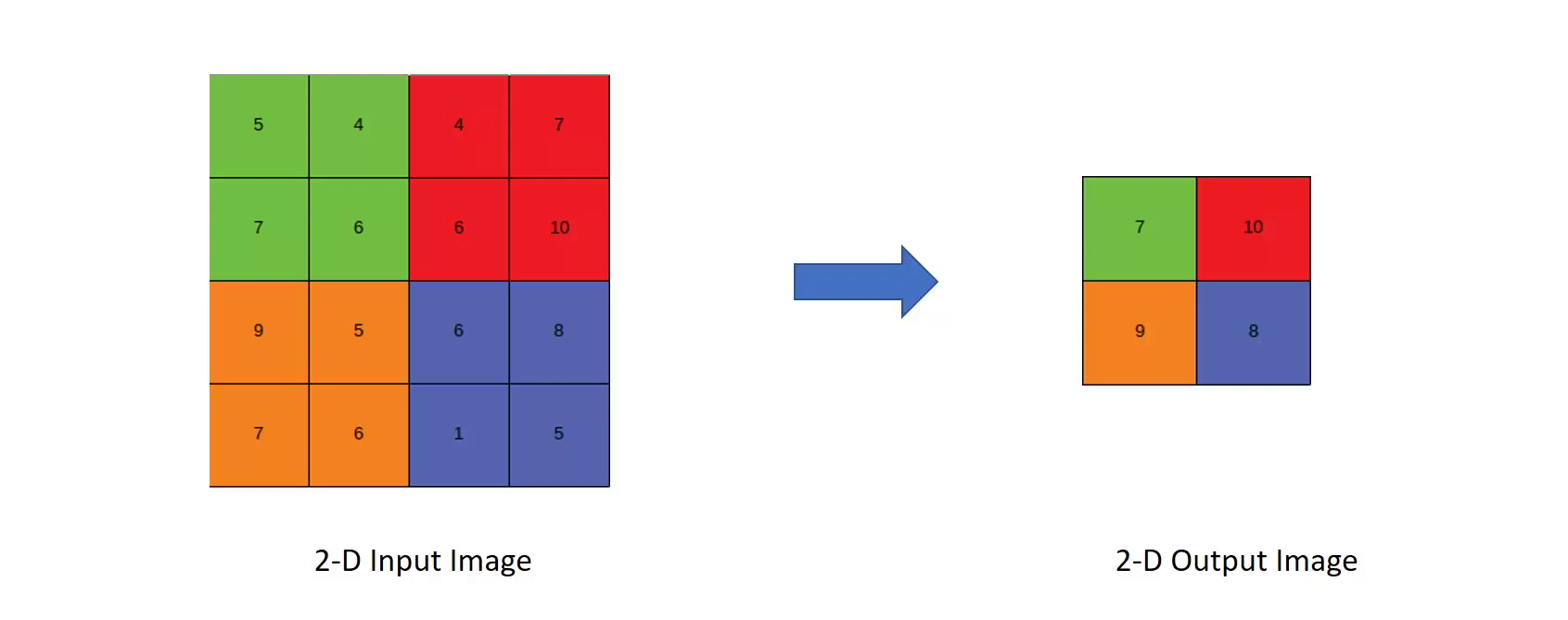Max Pooling

Now we can see that the dimension has been reduced from a 4 X 4 input to a 2 X 2 output. But how pooling extract the dominant features.

In the above image we took the max value of the filter window and if the filter has detected something then the maximum value represents those detections hence taking only that value only and throwing away the redundant information.

Now let’s see how pooling works with the 3d input image.

Consider the below image with a 3d input image having RGB channels. So, when we apply pooling to 3d image it works independently on each channel. The filter will first go through the red channel and takes the max values for each window and then similarly to the green and blue channels. So, for a 3d input we get a 3d output with reduced dimensions.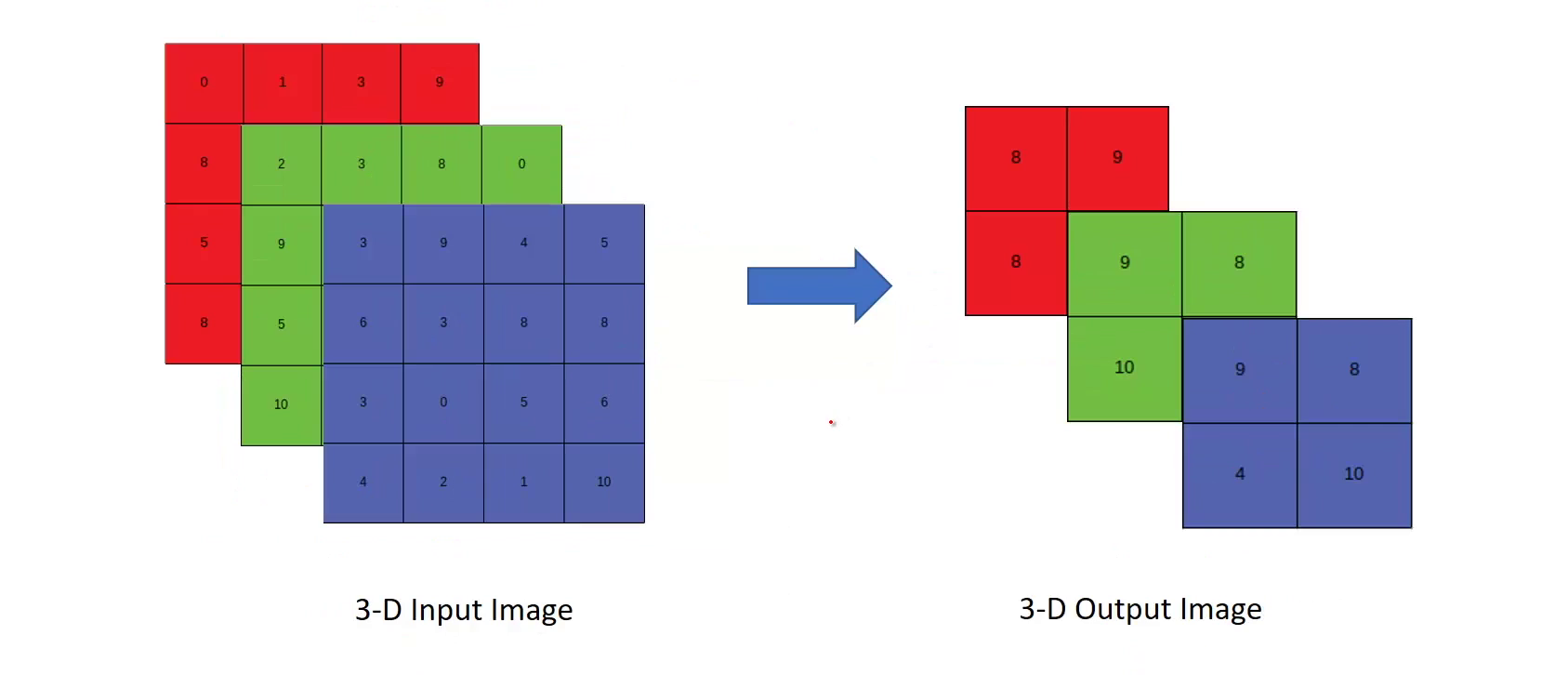Max Pooling on 3d image

Let’s take a look at different types of pooling

Max Pooling - we have already discussed this pooling where we take maximum value of the filter window.

Average pooling - It is similar in operation as max pooling the only difference is instead of taking the maximum of the values, we take the average of the values in the window.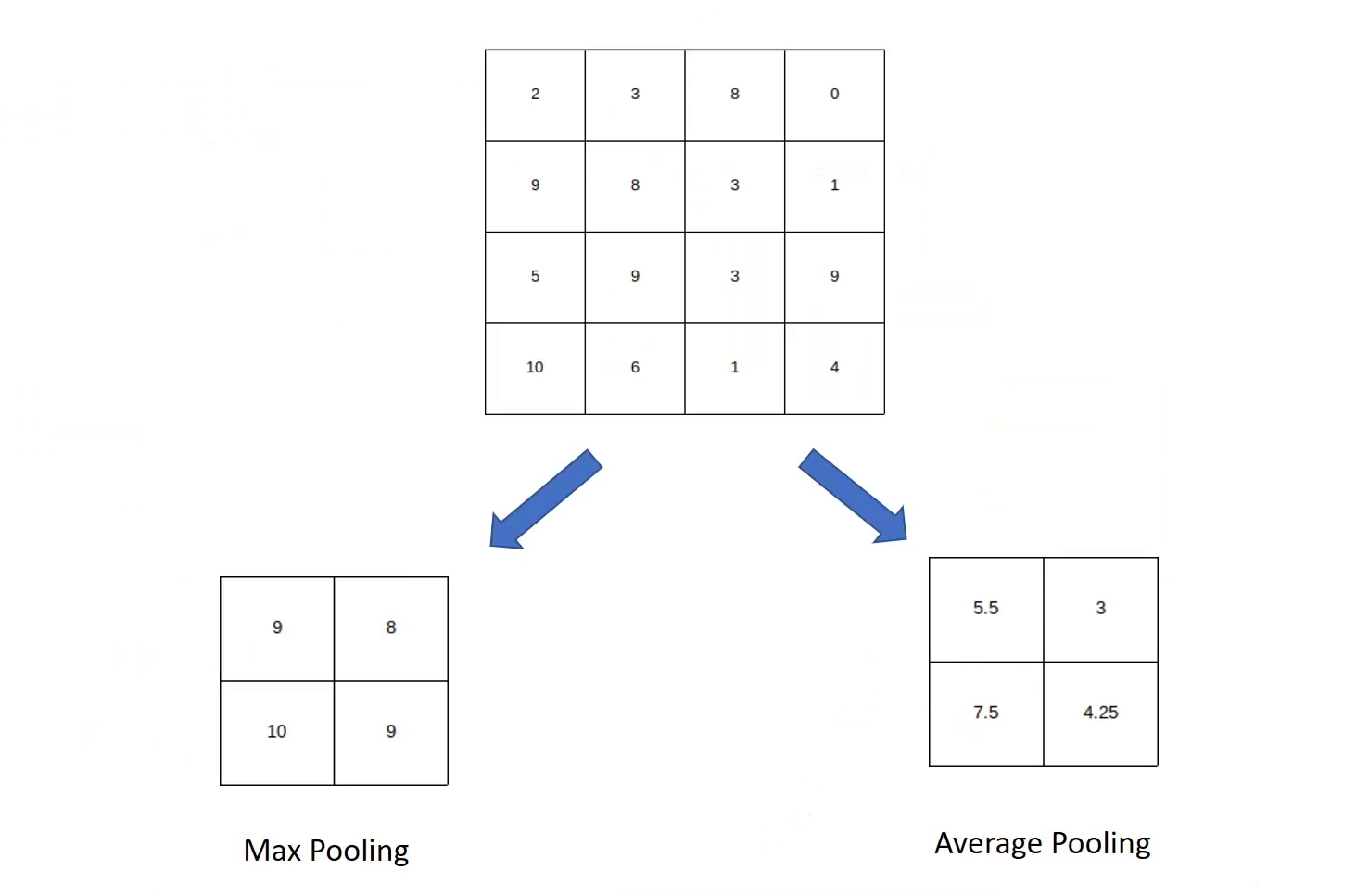Different types of pooling

Global average pooling

Earlier we have seen that if we have a 3D input image we will get a 3d output. But in global average pooling if we pass a 3D input, we get a 1D output. This is used when we want the CNN or the feature extraction part to connect to the fully connected part.

In global average pooling the filter size is equal to the size of the entire image. So instead to a 2 X 2 filter that we used in max pooling and average pooling the filter size will be 4 X 4 that is equal to the size of the images. It takes the average of the entire channel. For example, it will first take the average of entire red channel then green and then blue and convert it into a 1D vector.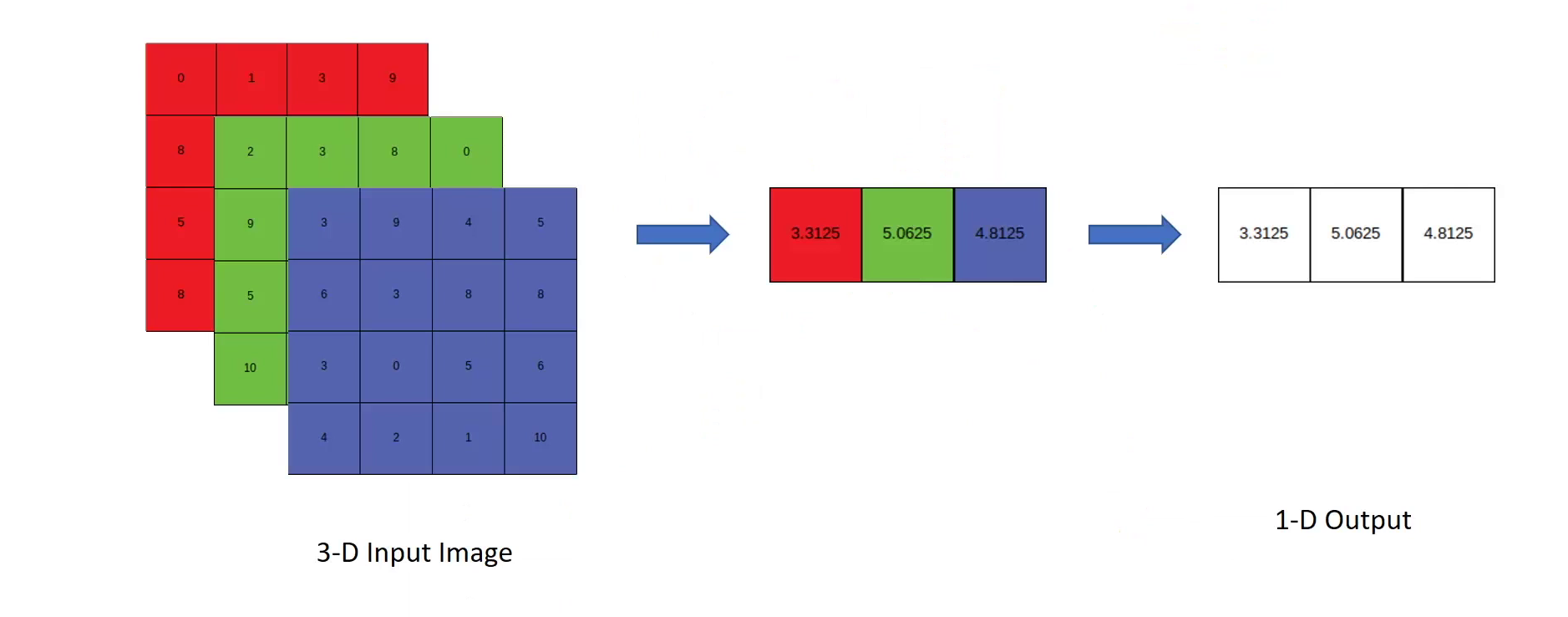Global average pooling

Global max pooling

It is similar to the global average pooling the only difference is that instead of taking average we take maximum value of the window.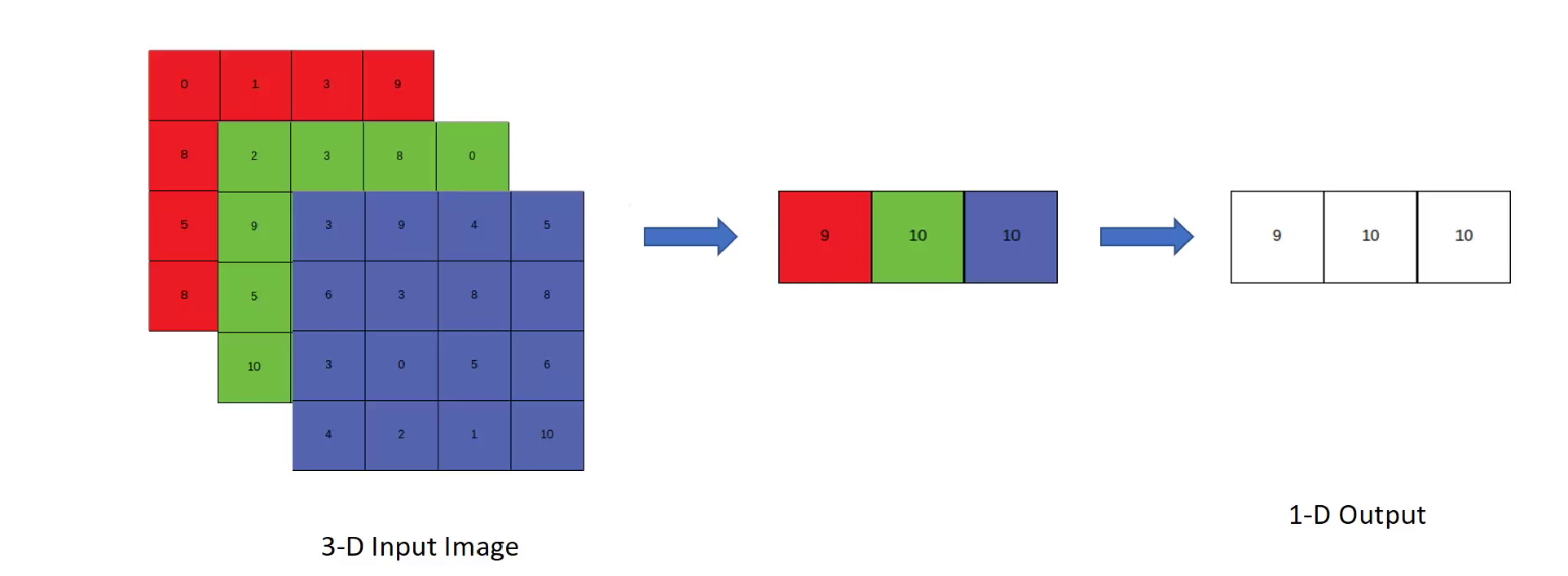Global max pooling

So this is it about pooling. I hope after reading this article you have got a good understanding of pooling layer in cnn. Check out my articles on the basics of convolution neural networkfilters, stride and padding.

Understanding mean Average Precision for Object Detection (with Python Code)

Photo by  Avel Chuklanov  on  Unsplash If you ever worked on object detection problem where you need to predict the bounding box coordinates of the objects, you may have come across the term mAP (mean average precision). mAP is a metric used for evaluating object detectors. As the name suggest it is the average of the AP. To understand mAP , first we need to understand what is precision, recall and IoU(Intersection over union). Almost everyone is familiar with first two terms, in case you don’t know these terms I am here to help you. Precision and Recall Precision: It tells us how accurate is our predictions or proportion of data points that our model says relevant are actually relevant. Formula for precision Recall: It is ability of a model to find all the data points of inte